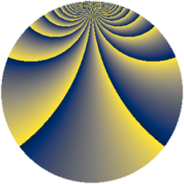# Properties

 Label 2736.2.ekLevel $2736$ Weight $2$ Character orbit 2736.ek Rep. character $\chi_{2736}(221,\cdot)$ Character field $\Q(\zeta_{12})$ Dimension $1904$ Sturm bound $960$

# Related objects

## Defining parameters

 Level: $$N$$ $$=$$ $$2736 = 2^{4} \cdot 3^{2} \cdot 19$$ Weight: $$k$$ $$=$$ $$2$$ Character orbit: $$[\chi]$$ $$=$$ 2736.ek (of order $$12$$ and degree $$4$$) Character conductor: $$\operatorname{cond}(\chi)$$ $$=$$ $$2736$$ Character field: $$\Q(\zeta_{12})$$ Sturm bound: $$960$$

## Dimensions

The following table gives the dimensions of various subspaces of $$M_{2}(2736, [\chi])$$.

Total New Old
Modular forms 1936 1936 0
Cusp forms 1904 1904 0
Eisenstein series 32 32 0

## Trace form

 $$1904q - 6q^{2} - 6q^{3} + 2q^{4} - 2q^{6} + O(q^{10})$$ $$1904q - 6q^{2} - 6q^{3} + 2q^{4} - 2q^{6} - 12q^{10} - 12q^{11} - 6q^{13} - 12q^{14} - 12q^{15} + 2q^{16} - 12q^{18} - 8q^{19} - 54q^{20} - 6q^{21} + 14q^{24} - 12q^{28} - 12q^{29} + 16q^{30} - 6q^{32} - 12q^{33} - 30q^{35} - 18q^{36} - 6q^{38} + 30q^{42} + 2q^{43} + 66q^{44} - 18q^{45} - 6q^{48} + 896q^{49} - 6q^{51} - 6q^{52} - 46q^{54} - 4q^{58} - 12q^{59} - 6q^{60} - 4q^{61} + 12q^{62} + 24q^{63} - 16q^{64} + 62q^{66} - 6q^{67} - 36q^{68} + 18q^{69} + 126q^{72} - 90q^{74} + 48q^{75} - 26q^{76} - 12q^{77} - 6q^{78} - 12q^{79} - 48q^{80} - 4q^{81} + 4q^{82} - 12q^{83} + 22q^{85} - 6q^{86} + 102q^{90} - 54q^{91} - 6q^{92} + 4q^{93} + 24q^{94} - 12q^{95} - 132q^{96} - 12q^{97} + 54q^{98} + 44q^{99} + O(q^{100})$$

## Decomposition of $$S_{2}^{\mathrm{new}}(2736, [\chi])$$ into newform subspaces

The newforms in this space have not yet been added to the LMFDB.